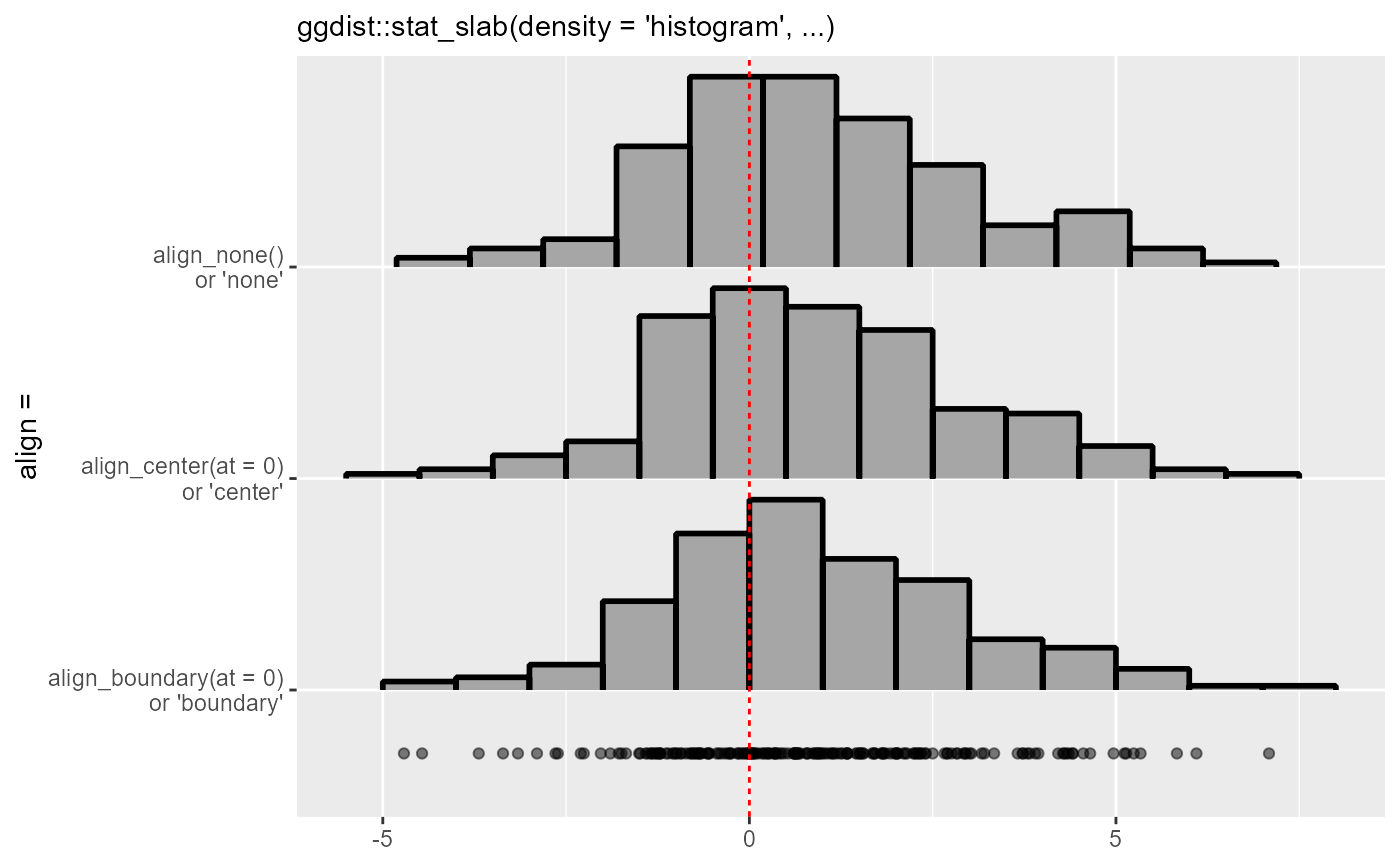Methods for aligning breaks (bins) in histograms, as used in the align argument to density_histogram(). Supports automatic partial function application.

align_none(breaks)

align_boundary(breaks, at = 0)

align_center(breaks, at = 0)

## Arguments

breaks

A sorted vector of breaks (bin edges).

at

A scalar numeric giving an alignment point.

• For align_boundary(): align breaks so that a bin edge lines up with at.

• For align_center(): align breaks so that the center of a bin lines up with at.

## Value

A scalar numeric returning an offset to be subtracted from breaks.

## Details

These functions take a sorted vector of equally-spaced breaks giving bin edges and return a numeric offset which, if subtracted from breaks, will align them as desired:

• align_none() performs no alignment (it always returns 0).

• align_boundary() ensures that a bin edge lines up with at.

• align_center() ensures that a bin center lines up with at.

For align_boundary() (respectively align_center()), if no bin edge (or center) in the range of breaks would line up with at, it ensures that at is an integer multiple of the bin width away from a bin edge (or center).

density_histogram(), breaks

## Examples

library(ggplot2)

set.seed(1234)
x = rnorm(200, 1, 2)

# If we manually specify a bin width using breaks_fixed(), the default
# alignment (align_none()) will not align bin edges to any "pretty" numbers.
# Here is a comparison of the three alignment methods on such a histogram:
ggplot(data.frame(x), aes(x)) +
stat_slab(
aes(y = "align_none()\nor 'none'"),
density = "histogram",
breaks = breaks_fixed(width = 1),
outline_bars = TRUE,
# no need to specify align; align_none() is the default
color = "black",
) +
stat_slab(
aes(y = "align_center(at = 0)\nor 'center'"),
density = "histogram",
breaks = breaks_fixed(width = 1),
align = align_center(at = 0),   # or align = "center"
outline_bars = TRUE,
color = "black",
) +
stat_slab(
aes(y = "align_boundary(at = 0)\nor 'boundary'"),
density = "histogram",
breaks = breaks_fixed(width = 1),
align = align_boundary(at = 0), # or align = "boundary"
outline_bars = TRUE,
color = "black",
) +
geom_point(aes(y = 0.7), alpha = 0.5) +
labs(
subtitle = "ggdist::stat_slab(density = 'histogram', ...)",
y = "align =",
x = NULL
) +
geom_vline(xintercept = 0, linetype = "22", color = "red")